DraftKings 2019 Pac-12 Odds
-139
0.72 to 1Utah
50.5% implied probability

+110
1.1 to 1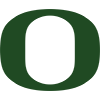Oregon
41.3% implied probability

+4000
40 to 1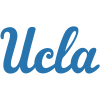UCLA
2.1% implied probability

+4000
40 to 1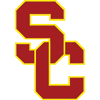USC
2.1% implied probability

+5000
50 to 1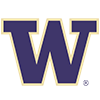Washington
1.7% implied probability

+10000
100 to 1Stanford
0.9% implied probability

+25000
250 to 1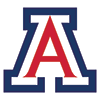Arizona
0.3% implied probability

+25000
250 to 1Arizona State
0.3% implied probability

+50000
500 to 1Oregon State
0.2% implied probability

+50000
500 to 1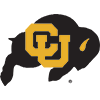Colorado
0.2% implied probability

+50000
500 to 1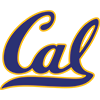California
0.2% implied probability

+50000
500 to 1Washington State
0.2% implied probability